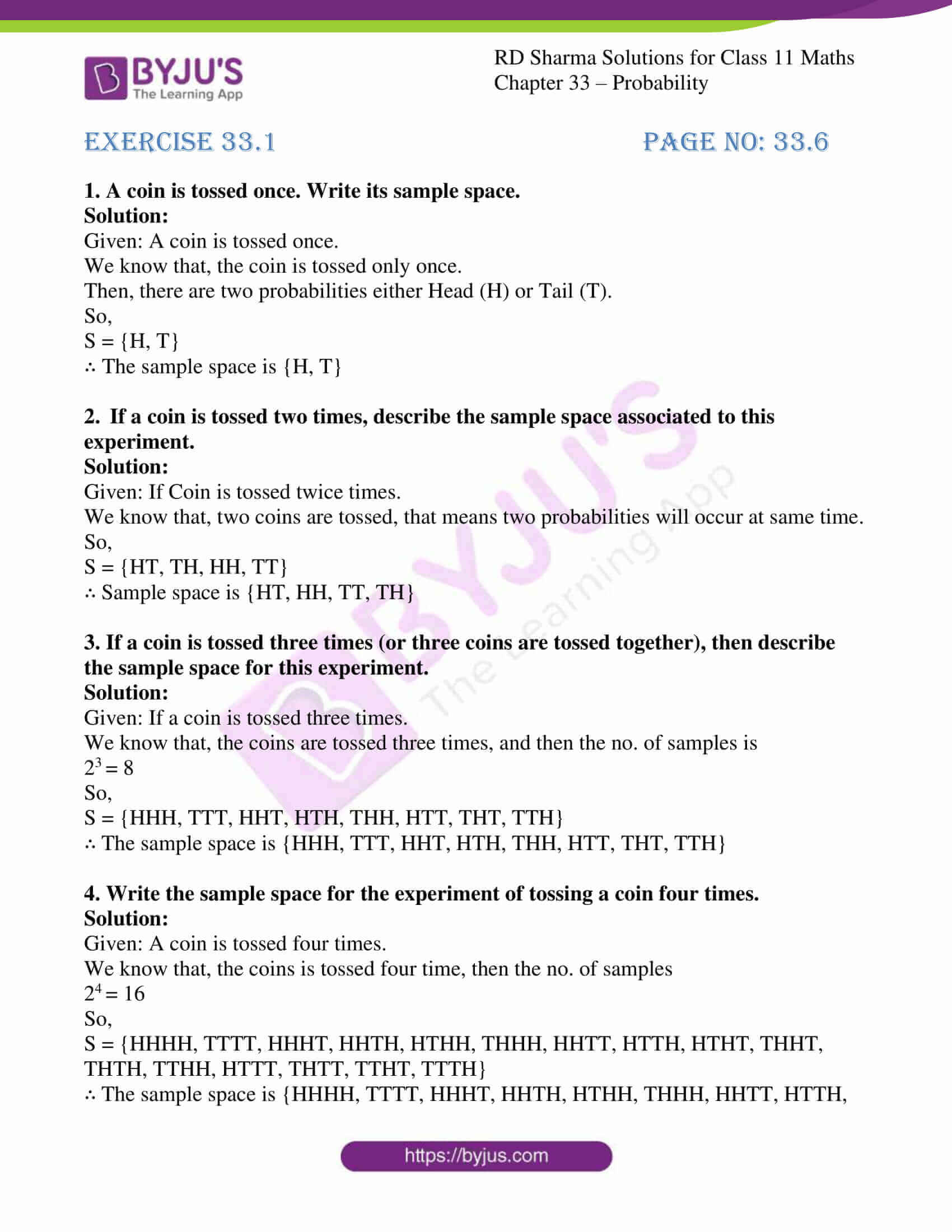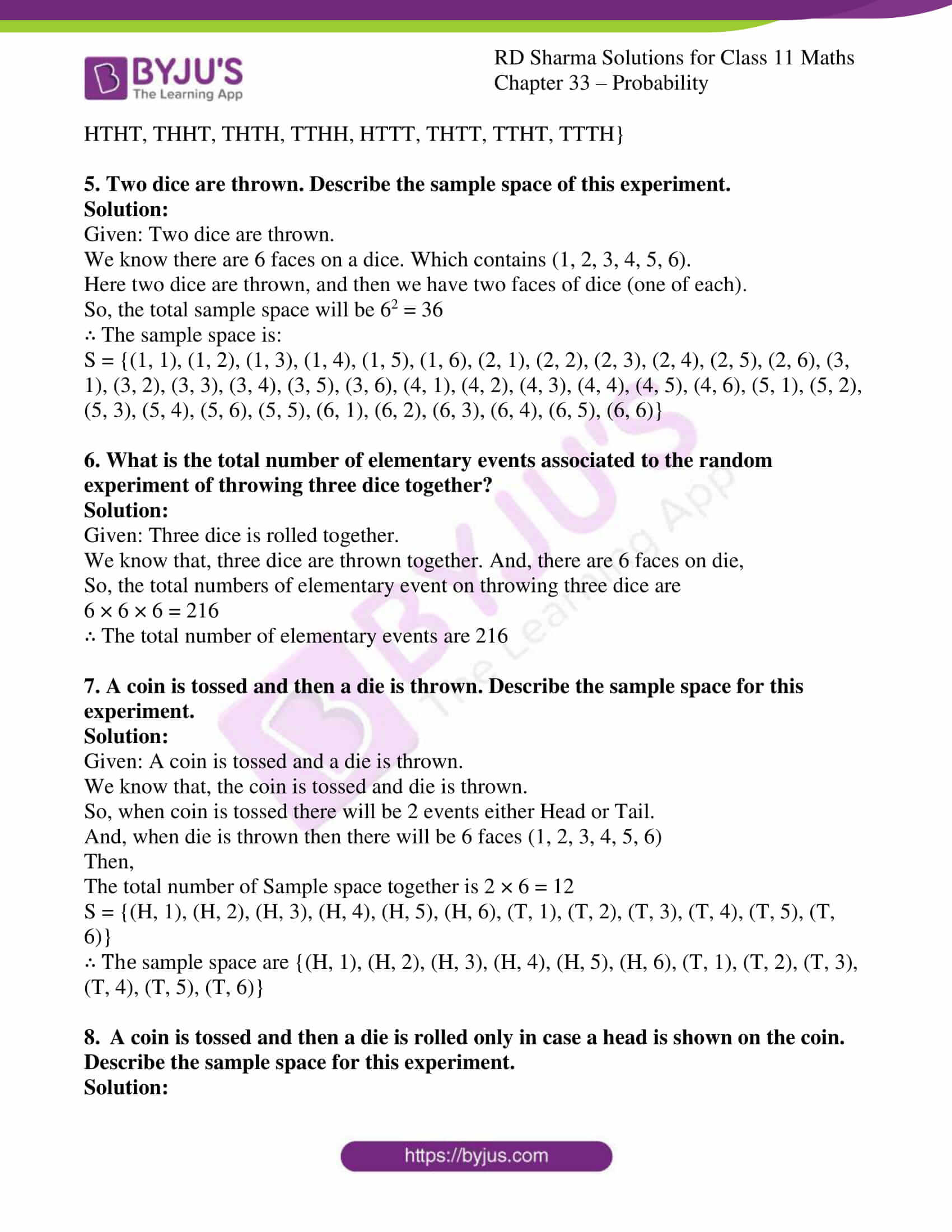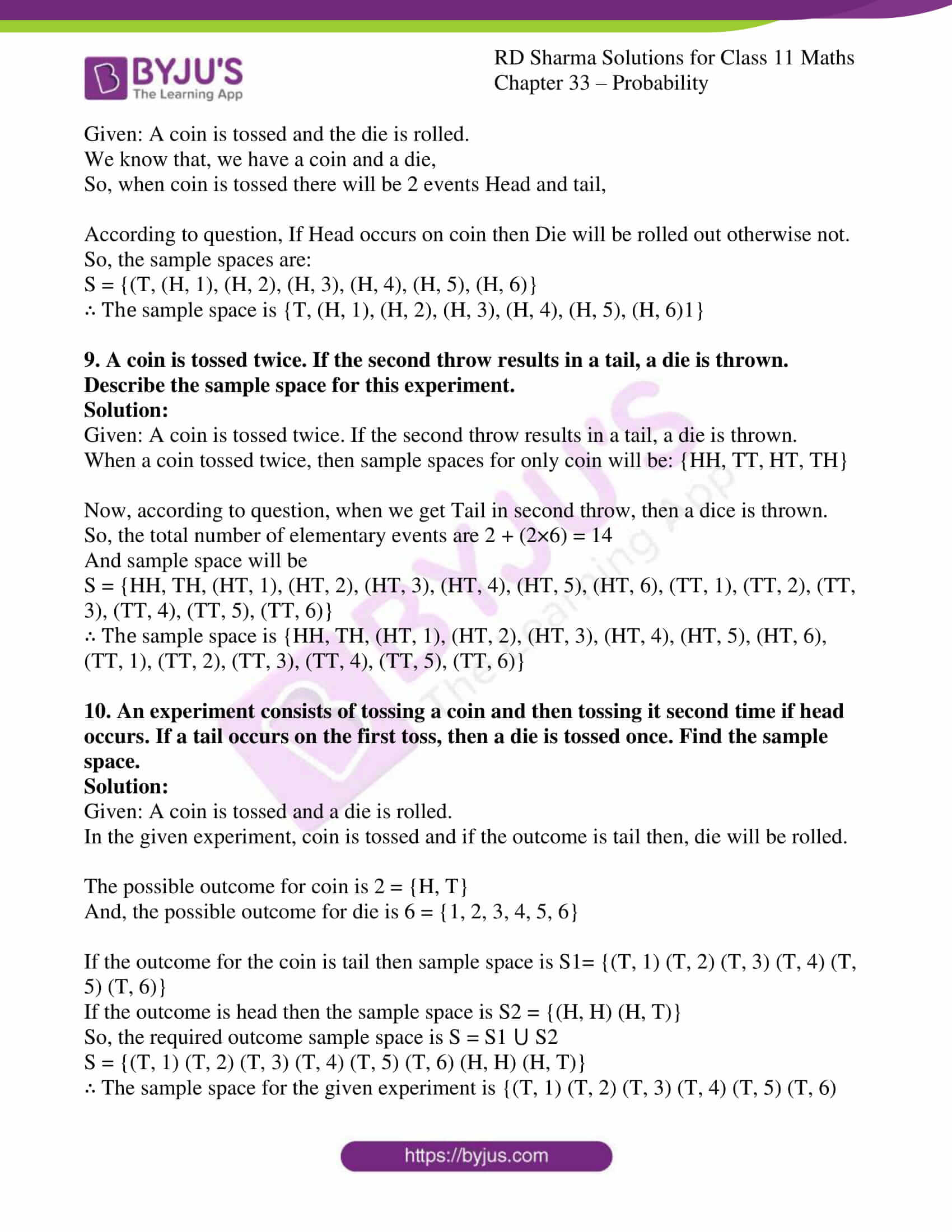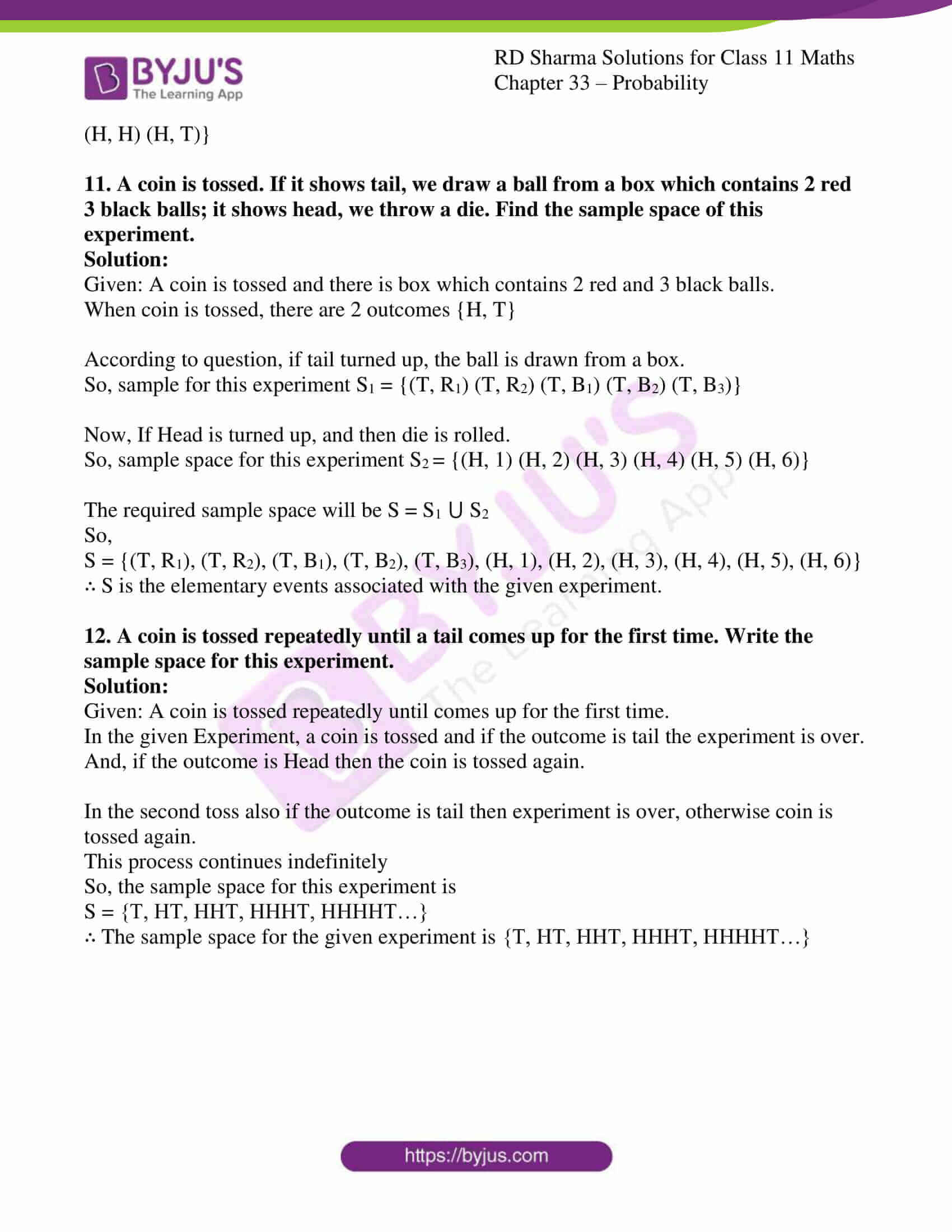# RD Sharma Solutions for Class 11 Chapter 33 - Probability Exercise 33.1

The word experiment means an operation, which can produce some well-defined outcomes. There are two types of experiments (i) Deterministic experiments and (ii) Random or Probability experiments. In this exercise, we shall discuss the above experiments with suitable examples. Numerous solved examples are present before the exercise problems to provide a clear understanding among students. Students can self analyse their weaknesses and work on them to yield good results in the board exams.

Students can refer to RD Sharma Class 11 Maths Solutions and download the free pdf, from the links given below.

## Download the pdf of RD Sharma Solutions for Class 11 Maths Exercise 33.1 Chapter 33 – Probability### Access answers to RD Sharma Solutions for Class 11 Maths Exercise 33.1 Chapter 33 – Probability

#### EXERCISE 33.1 PAGE NO: 33.6

1. A coin is tossed once. Write its sample space.

Solution:

Given: A coin is tossed once.

We know that, the coin is tossed only once.

Then, there are two probabilities either Head (H) or Tail (T).

So,

S = {H, T}

∴ The sample space is {H, T}

2. If a coin is tossed two times, describe the sample space associated to this experiment.

Solution:

Given: If Coin is tossed twice times.

We know that, two coins are tossed, that means two probabilities will occur at same time.

So,

S = {HT, TH, HH, TT}

∴ Sample space is {HT, HH, TT, TH}

3. If a coin is tossed three times (or three coins are tossed together), then describe the sample space for this experiment.

Solution:

Given: If a coin is tossed three times.

We know that, the coins are tossed three times, and then the no. of samples is

23 = 8

So,

S = {HHH, TTT, HHT, HTH, THH, HTT, THT, TTH}

∴ The sample space is {HHH, TTT, HHT, HTH, THH, HTT, THT, TTH}

4. Write the sample space for the experiment of tossing a coin four times.

Solution:

Given: A coin is tossed four times.

We know that, the coins is tossed four time, then the no. of samples

24 = 16

So,

S = {HHHH, TTTT, HHHT, HHTH, HTHH, THHH, HHTT, HTTH, HTHT, THHT, THTH, TTHH, HTTT, THTT, TTHT, TTTH}

∴ The sample space is {HHHH, TTTT, HHHT, HHTH, HTHH, THHH, HHTT, HTTH, HTHT, THHT, THTH, TTHH, HTTT, THTT, TTHT, TTTH}

5. Two dice are thrown. Describe the sample space of this experiment.

Solution:

Given: Two dice are thrown.

We know there are 6 faces on a dice. Which contains (1, 2, 3, 4, 5, 6).

Here two dice are thrown, and then we have two faces of dice (one of each).

So, the total sample space will be 62 = 36

∴ The sample space is:

S = {(1, 1), (1, 2), (1, 3), (1, 4), (1, 5), (1, 6), (2, 1), (2, 2), (2, 3), (2, 4), (2, 5), (2, 6), (3, 1), (3, 2), (3, 3), (3, 4), (3, 5), (3, 6), (4, 1), (4, 2), (4, 3), (4, 4), (4, 5), (4, 6), (5, 1), (5, 2), (5, 3), (5, 4), (5, 6), (5, 5), (6, 1), (6, 2), (6, 3), (6, 4), (6, 5), (6, 6)}

6. What is the total number of elementary events associated to the random experiment of throwing three dice together?

Solution:

Given: Three dice is rolled together.

We know that, three dice are thrown together. And, there are 6 faces on die,

So, the total numbers of elementary event on throwing three dice are

6 × 6 × 6 = 216

∴ The total number of elementary events are 216

7. A coin is tossed and then a die is thrown. Describe the sample space for this experiment.

Solution:

Given: A coin is tossed and a die is thrown.

We know that, the coin is tossed and die is thrown.

So, when coin is tossed there will be 2 events either Head or Tail.

And, when die is thrown then there will be 6 faces (1, 2, 3, 4, 5, 6)

Then,

The total number of Sample space together is 2 × 6 = 12

S = {(H, 1), (H, 2), (H, 3), (H, 4), (H, 5), (H, 6), (T, 1), (T, 2), (T, 3), (T, 4), (T, 5), (T, 6)}

∴ The sample space are {(H, 1), (H, 2), (H, 3), (H, 4), (H, 5), (H, 6), (T, 1), (T, 2), (T, 3), (T, 4), (T, 5), (T, 6)}

8. A coin is tossed and then a die is rolled only in case a head is shown on the coin. Describe the sample space for this experiment.

Solution:

Given: A coin is tossed and the die is rolled.

We know that, we have a coin and a die,

So, when coin is tossed there will be 2 events Head and tail,

According to question, If Head occurs on coin then Die will be rolled out otherwise not.

So, the sample spaces are:

S = {(T, (H, 1), (H, 2), (H, 3), (H, 4), (H, 5), (H, 6)}

∴ The sample space is {T, (H, 1), (H, 2), (H, 3), (H, 4), (H, 5), (H, 6)1}

9. A coin is tossed twice. If the second throw results in a tail, a die is thrown. Describe the sample space for this experiment.

Solution:

Given: A coin is tossed twice. If the second throw results in a tail, a die is thrown.

When a coin tossed twice, then sample spaces for only coin will be: {HH, TT, HT, TH}

Now, according to question, when we get Tail in second throw, then a dice is thrown.

So, the total number of elementary events are 2 + (2×6) = 14

And sample space will be

S = {HH, TH, (HT, 1), (HT, 2), (HT, 3), (HT, 4), (HT, 5), (HT, 6), (TT, 1), (TT, 2), (TT, 3), (TT, 4), (TT, 5), (TT, 6)}

∴ The sample space is {HH, TH, (HT, 1), (HT, 2), (HT, 3), (HT, 4), (HT, 5), (HT, 6), (TT, 1), (TT, 2), (TT, 3), (TT, 4), (TT, 5), (TT, 6)}

10. An experiment consists of tossing a coin and then tossing it second time if head occurs. If a tail occurs on the first toss, then a die is tossed once. Find the sample space.

Solution:

Given: A coin is tossed and a die is rolled.

In the given experiment, coin is tossed and if the outcome is tail then, die will be rolled.

The possible outcome for coin is 2 = {H, T}

And, the possible outcome for die is 6 = {1, 2, 3, 4, 5, 6}

If the outcome for the coin is tail then sample space is S1= {(T, 1) (T, 2) (T, 3) (T, 4) (T, 5) (T, 6)}

If the outcome is head then the sample space is S2 = {(H, H) (H, T)}

So, the required outcome sample space is S = S1 ⋃ S2

S = {(T, 1) (T, 2) (T, 3) (T, 4) (T, 5) (T, 6) (H, H) (H, T)}

∴ The sample space for the given experiment is {(T, 1) (T, 2) (T, 3) (T, 4) (T, 5) (T, 6) (H, H) (H, T)}

11. A coin is tossed. If it shows tail, we draw a ball from a box which contains 2 red 3 black balls; it shows head, we throw a die. Find the sample space of this experiment.

Solution:

Given: A coin is tossed and there is box which contains 2 red and 3 black balls.

When coin is tossed, there are 2 outcomes {H, T}

According to question, if tail turned up, the ball is drawn from a box.

So, sample for this experiment S1 = {(T, R1) (T, R2) (T, B1) (T, B2) (T, B3)}

Now, If Head is turned up, and then die is rolled.

So, sample space for this experiment S2 = {(H, 1) (H, 2) (H, 3) (H, 4) (H, 5) (H, 6)}

The required sample space will be S = S1 ⋃ S2

So,

S = {(T, R1), (T, R2), (T, B1), (T, B2), (T, B3), (H, 1), (H, 2), (H, 3), (H, 4), (H, 5), (H, 6)}

∴ S is the elementary events associated with the given experiment.

12. A coin is tossed repeatedly until a tail comes up for the first time. Write the sample space for this experiment.

Solution:

Given: A coin is tossed repeatedly until comes up for the first time.

In the given Experiment, a coin is tossed and if the outcome is tail the experiment is over.

And, if the outcome is Head then the coin is tossed again.

In the second toss also if the outcome is tail then experiment is over, otherwise coin is tossed again.

This process continues indefinitely

So, the sample space for this experiment is

S = {T, HT, HHT, HHHT, HHHHT…}

∴ The sample space for the given experiment is {T, HT, HHT, HHHT, HHHHT…}